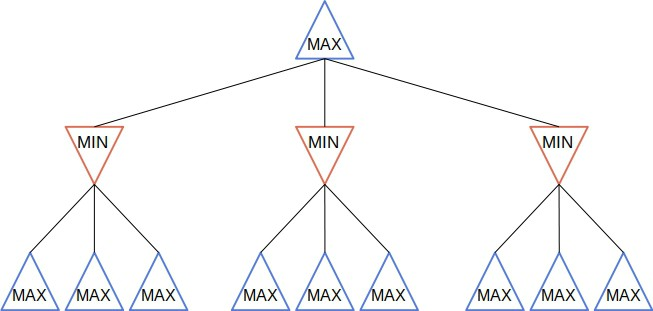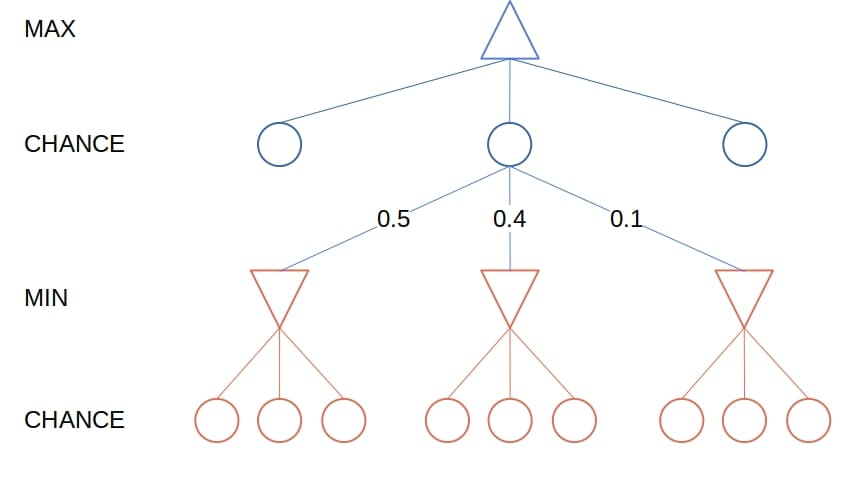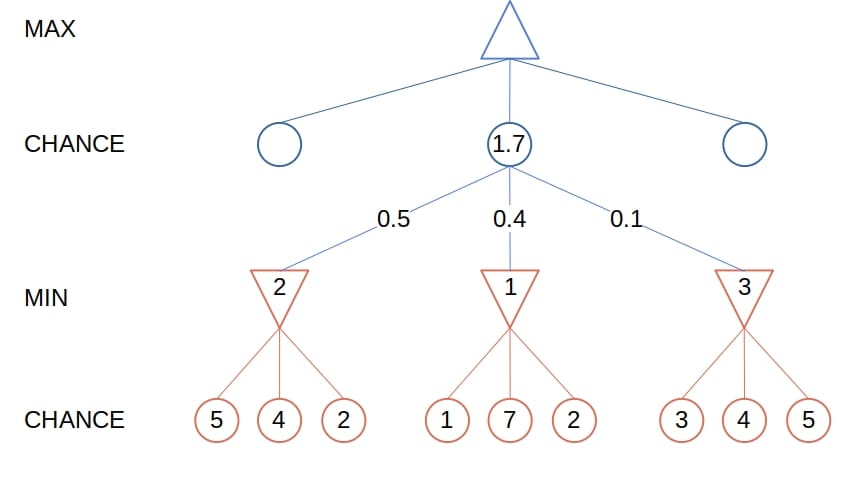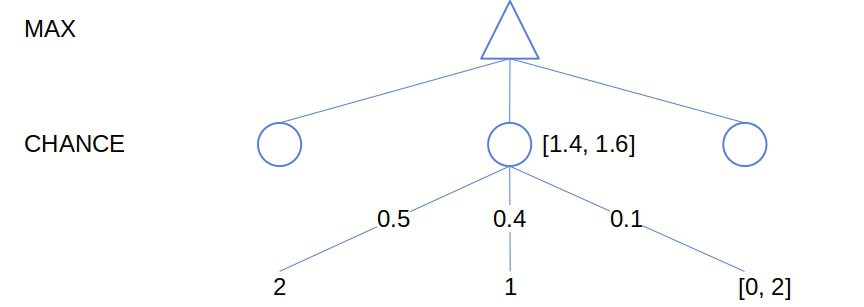## 1. Introduction

In this tutorial, we’ll present Expectimax, an adversarial search algorithm suitable for playing non-deterministic games.

In particular, we’ll focus on stochastic two-player games, which include random elements, such as the throwing of dice. However, since Expectimax is a modification of Minimax, the algorithm for playing deterministic games, we’ll first introduce the latter.

In classical search, an AI agent looks for the optimal sequence of actions to achieve some goal. The agent is the only entity in its environment that can take action, so all the changes come through its actions. Since it’s the only one there, the agent models only its state of belief about the environment. Then, the sequence of actions becomes a path between the states.

In adversarial search, the agent still searches for the optimal sequence of actions, but the environment is different. In contrast to classical search, it’s not alone here. There is at least one other AI (or human) agent that can act in their shared environment. Furthermore, the agents have conflicting goals, so they compete with one another. So, the optimal sequence the agents want to find is the one that leads to victory over the opponents.

The agents, therefore, model not only their states but also the states of all the other agents they compete with. Good examples of adversarial search are two-player or multiplayer games such as chess and poker.

## 3. Games

In AI game playing, we often refer to the states as positions and actions as moves. Just as it’s the case with general problems in classical search, the definition of a game includes multiple components:

• start position: it specifies the initial state of the game before the very first move
•: a function that tells us which player (agent) is to make its move in the state•: a function returning all the moves legal in the state•: the transition model specifying which state we get in by applying actionin the state•: a function that identifies the states in which the game is over
•: the utility function assigning a numerical value to playerin terminal stateThis article will focus on the games in which the utilities for all the players in each terminal state sum up to a constant. That constant is 0 most of the time, which is why we call such games “zero-sum”, although “constant-sum games” would be a more precise term. So, the players try to win the game by maximizing their utility at the end of it.

## 4. Deterministic Games

In deterministic games, there is no uncertainty about the environment, the actions possible to take in a state, and the state we get after applying an action. Therefore, all the components in the game definition are completely deterministic.

For instance, tic-tac-toe is a deterministic game. For any board state, we know without any doubt whose turn it is to play, which cells the player can mark, and what the board will look like after each possible move.

To play two-player games that are both deterministic and zero-sum, we use the algorithm known as Minimax.

### 4.1. Minimax

The two players go by names MAX and MIN. MAX is the player our AI agent runs Minimax for, while MIN is the opponent. MAX chooses the moves to maximize its utility, whereas MIN tries to minimize the result of MAX. Since the game is zero-sum, MIN maximizes its utility by minimizing that of MAX.

The search tree starts in the current state (which isinitially) and branches out by all the possible move sequences. A nodein the tree points to the state the agent gets to by following the path of moves fromto.

So, similar to classical search, a nodeis a structure representing a path to its stateand contains a pointer to its parent (). But, to keep things simple, we’ll consider that the functionsthroughin the game definition in Section 3 operate on nodes as well.

Minimax explores the tree by simulating the game and calculating the minimax value of each node:Starting from the nodewhen it’s the MAX’s turn to play, Minimax chooses the move which leads to the child with the highest minimax value.  Let’s say thatis that child. Then, MIN makes its move by running its instance of Minimax on, minimizing the utility of MAX. After that, it’s again MAX’s turn to make a move, so it starts from, and so on:### 4.2. Pseudocode

This is the pseudocode of Minimax:So, Minimax traverses the whole game tree to the leaves and returns the best move for the player at hand. If the tree is too deep,  we cut off the search at some depth, making non-terminal nodes our new leaves. Then, we evaluate the leaves by estimating their minimax value instead of calculating the utilities of the actual leaves and propagating them upward. We can also prune the tree with the alpha-beta search.

Evaluation functions we use for estimation should return numeric values that correlate with the actual minimax values. They should also be computationally lightweight and easy to implement. But, if it’s hard to design a quality evaluation function, we can use Monte Carlo Tree Search (MCTS) to evaluate a node.

## 5. Expectimax

Unlike deterministic games, the stochastic ones have at least one probabilistic element. For example,may return different sets of legal actions each time we call it, depending on the outcome of some random event such as the throwing of dice. Or, the result of an action can lead to multiple states, each with a certain probability.

For instance, we roll dice in a game of backgammon before each move. The numbers we get determine what moves we can make. MAX doesn’t know in advance what moves it will choose from at any point in the game, and the same goes for MIN’s actions. All the AI agent has at those chance branching points is a probability distribution over possible moves.

### 5.1. Chance Nodes

So, the search tree of stochastic games has an additional node type: the chance nodes. A chance noderepresents a probability distribution over the set of moves available to the player whose node is the parent of:Instead of the minimax values, the nodes have the expectimax values. They’re the same as the minimax values for MIN and MAX nodes, but for a chance node, the expectimax value is the expected value of its children:Or, as a recurrence:is an outcome of the random event at chance nodes, andis its probability.

### 5.2. Pseudocode

The ExpectiMax algorithm is very similar to MiniMax:Still, we should note two things. The first one is that the functionsanddon’t call one another like they do in Minimax. Instead, both call.

The second thing is that ifis a chance node, then there isn’t a single move to return. Its expectimax value is the expectation over the outcomes of all the possible moves. We pair it with, the empty move, for consistency with other cases.

### 5.3. Cut-offs and Evaluation Functions

Similar to what we did with Minimax trees, we’ll most probably have to limit the depth of the Expectimax trees by cutting off the search at some point. However, we’ll have to pay special care to design the evaluation functions that estimate non-terminal leaf nodes.

The evaluation functions in Minimax need only be correlated with the probabilities of winning. More precisely, they should preserve the order of moves. For example, let movelead to statein which the player haschance to win, and letlead to the statewhere the probability is. Then, Minimax requires only that evaluation functionassigns a higher value to:so thatcan use it to win.

However, in Expectimax, the evaluation functionshould be a positive linear transformation of the probabilities of winning. Thus, even if the order remains the same, small changes to the evaluation values can lead the agent to choose a different move.

If designing a good evaluation function proves to be too challenging, we can opt for MCTS.

### 5.4. Pruning

We can apply the alpha-beta pruning technique to Expectimax even though it may come as counter-intuitive at first sight. The goal of pruning is to prove that we don’t need to consider certain moves so that we completely disregard the sub-trees rooted in the nodes those moves lead us to. However, the expectimax values require visiting all the children of a chance node and calculating their expectimax values. So, it appears that pruning is incompatible with Expectimax.

But, there’s a way to circumvent this issue. If we bound the range of the evaluation function from below and above, we can determine the bounds of the expectimax value of a chance node even if we don’t evaluate all its children and their sub-trees:## 6. Conclusion

In this article, we presented the Expectimax algorithm for stochastic games. We derived it from Minimax and talked about two optimization strategies: limiting the game tree depth and pruning the search tree.